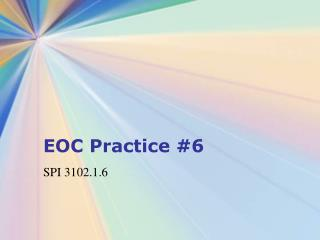DownloadDownload PresentationEOC Practice #6

# EOC Practice #6

Télécharger la présentation## EOC Practice #6

- - - - - - - - - - - - - - - - - - - - - - - - - - - E N D - - - - - - - - - - - - - - - - - - - - - - - - - - -
##### Presentation Transcript

1. EOC Practice #6 SPI 3102.1.6

2. EOC Practice #6 • Determine and interpret slope in multiple contexts including rate of change in real-world problems.

3. Find the slope of the line that passes through (–6, 1) and (4, –3). • C. • – D. –

4. Brandon works in a shoe store. His • daily earnings, y, are represented by the equation y = 20x + 75 based on selling x pairs of shoes. What is represented by the slope in this equation? • The total pairs of shoes Brandon sells • each day • The total amount of money Brandon earns • each day • The amount of money Brandon earns for • each pair of shoes he sells • The amount of money Brandon earns each • day, even if he sells no shoes

5. The distance in miles, y, a rower in a • canoe is from the dock after rowing x hours is represented by the equation y = 5x + 11. What does the slope represent in this situation? • The speed of the current • The speed of the rower/canoe • The distance the rower is from the dock when x = 0 • The average speed of the oar as it • passes through the water

6. What is the slope of the line • 3x – 7y = 11? • – C. 3 • B. D. –3

7. In 1991, the federal minimum wage rate • was \$4.25 per hour. In 1997, it was increased to \$5.15. Find the annual rate of change in the federal minimum wage rate from 1991 to 1997. • \$0.15 per year • \$0.18 per year • \$0.55 per year • D. \$0.90 per year

8. 6. The table below shows the amount spent on food and drink at U.S. restaurants in recent years. Find the rate of change for 1980-1990. • 13.7 billion per year • 12.8 billion per year • 10 billion per year • D. 11.9 billion per year